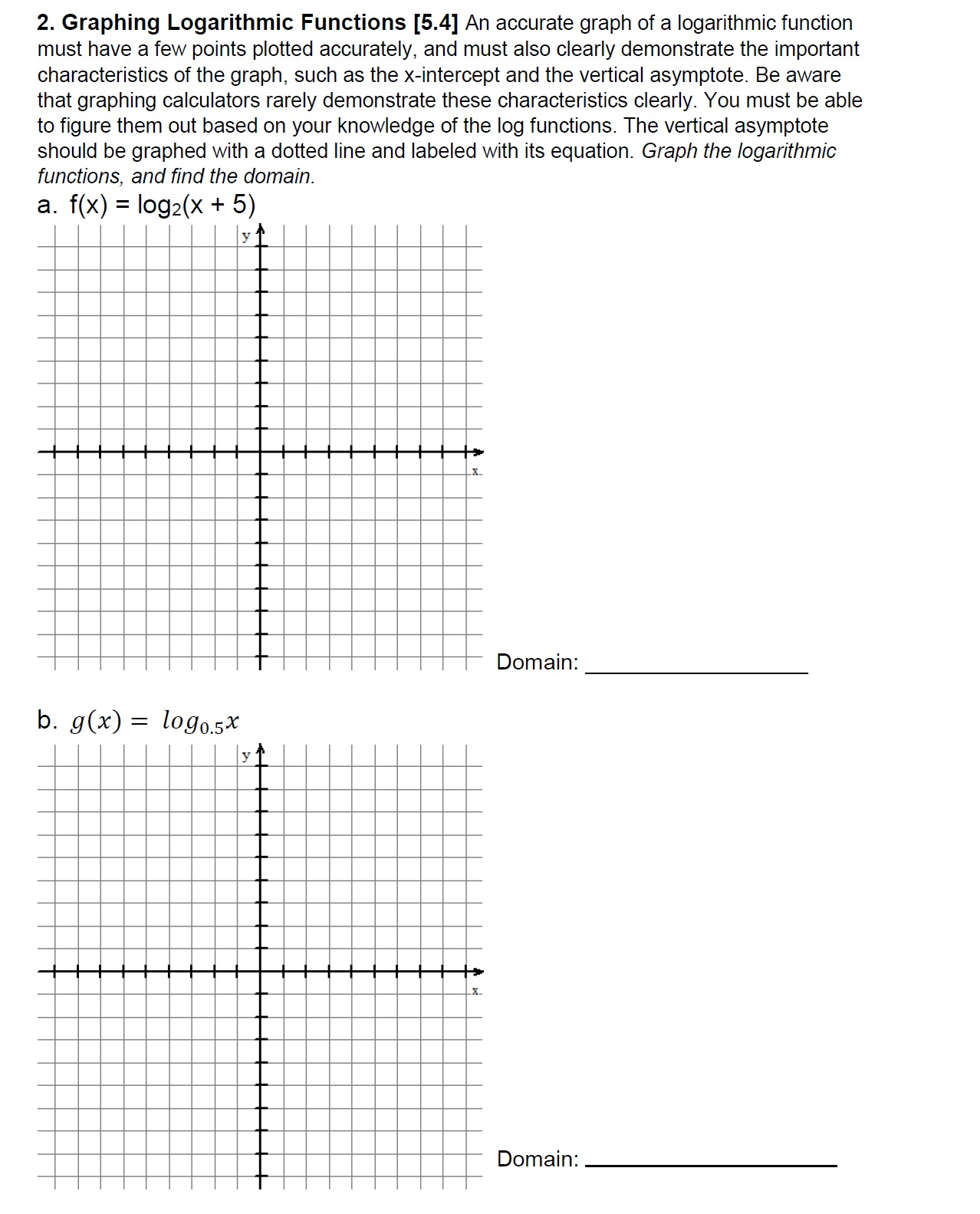# 2. Graphing Logarithmic Functions ⑤41 An accurate graph of a logarithmic functionmust have a few points plotted accurately, and must also clearly demonstrate the importantcharacteristics of the graph, such as the x-intercept and the vertical asymptote. Be awarethat graphing calculators rarely demonstrate these characteristics clearly. You must be ableto figure them out based on your knowledge of the log functions. The vertical asymptoteshould be graphed with a dotted line and labeled with its equation. Graph the logarithmicfunctions, and find the domaina. f(x) - log2(X + 5)22X.Domain.Domain:

Question
33 viewshelp_outlineImage Transcriptionclose2. Graphing Logarithmic Functions ⑤41 An accurate graph of a logarithmic function must have a few points plotted accurately, and must also clearly demonstrate the important characteristics of the graph, such as the x-intercept and the vertical asymptote. Be aware that graphing calculators rarely demonstrate these characteristics clearly. You must be able to figure them out based on your knowledge of the log functions. The vertical asymptote should be graphed with a dotted line and labeled with its equation. Graph the logarithmic functions, and find the domain a. f(x) - log2(X + 5) 2 2 X. Domain. Domain: fullscreen
check_circle

Step 1

To draw the graph the logarithmic function f(x)=log2(x+5) and g(x)=log0.5x and find its domain also draw the x-intercept and vertical asymptotes. Now, first find the domain of the function then plot vertical asymptotes and finally draw the graph.

Step 2

Now, for the domain of the function f(x)=log2(x+5) and g(x)=log0.5x. The domain of the function is value where the function defined and possess real value.

Step 3

Now, for the asymptotes of the function f(x)=log2(x+5) and g(x)=log0.5x. Compare both function with standard...

### Want to see the full answer?

See Solution

#### Want to see this answer and more?

Solutions are written by subject experts who are available 24/7. Questions are typically answered within 1 hour.*

See Solution
*Response times may vary by subject and question.
Tagged in

### Logarithms